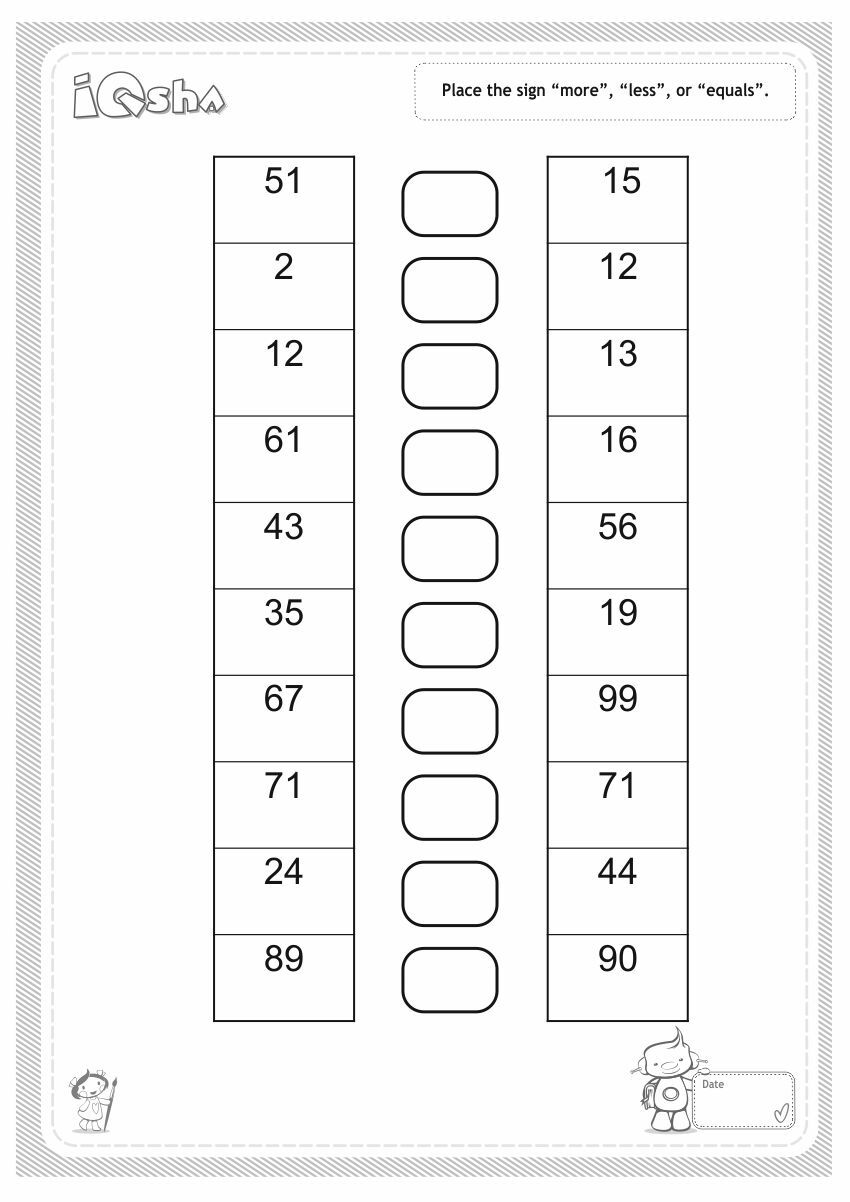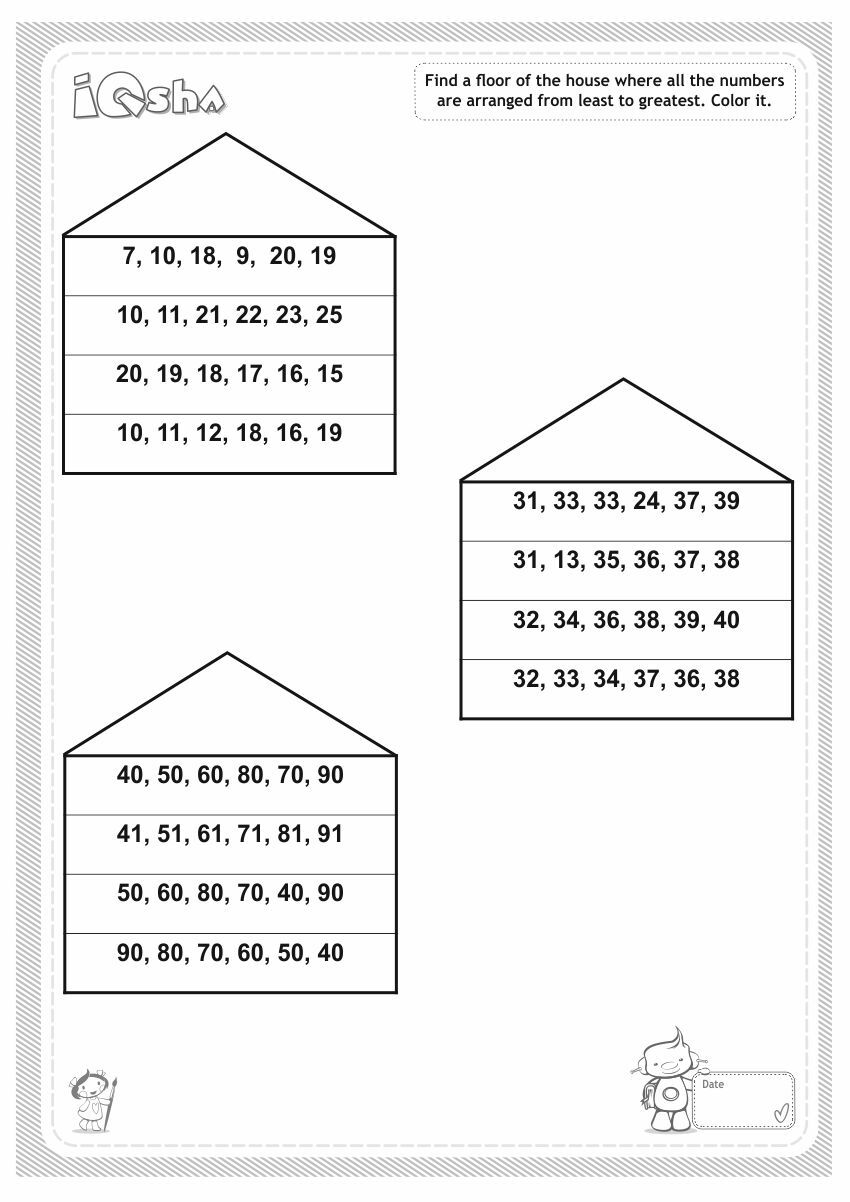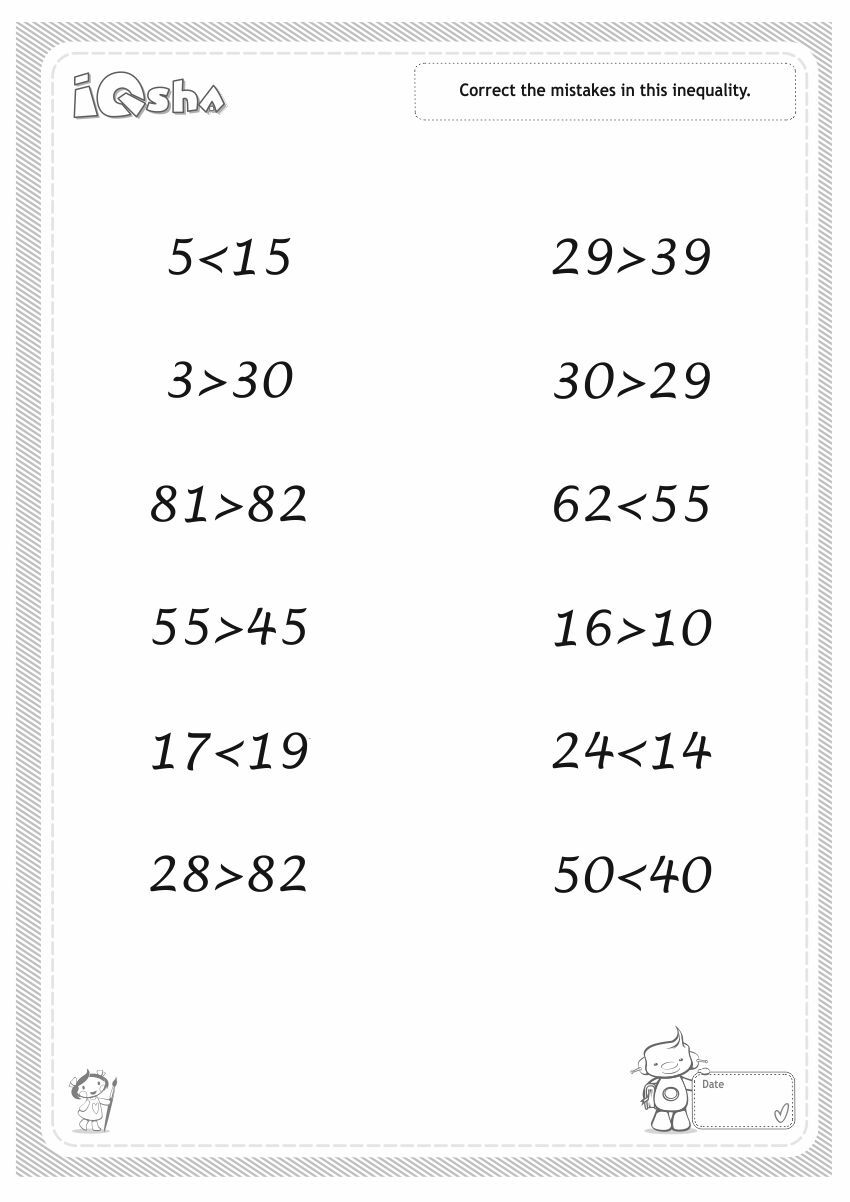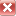# Comparing Two-Digit Numbers

19/06/2014, 12:42

If your child understands how two-digit numbers are formed and can count to 100, he can easily compare two-digit numbers.How to compare from 0 to 99

1. Two-digit numbers always consist of at least one ten. 10 is the smallest two-digit number. When comparing any single digit with a two-digit one, the two-digit will always be greater.
2. 2. Usually we compare 2 two-digit numbers using the highest category – the first figure. The first figure designates the number of tens. When we compare the numbers 45 and 57, we begin by comparing the first figures – the number of tens. In the number 45 there are 4 tens (the first figure is 4), and in the number 57 there are five tens (the first figure is 5).Since 4 < 5, that means 45 < 57. The subsequent figures are not important here.
3. 3. If the number of tens in the numbers is the same (the first figures are equal), we compare the quantity of the second figure. We will compare numbers 45 and 46. These numbers have an equal number of tens - four. As the number of tens is identical, we will compare the numbers of the second digits. In the number 45 there are five ones, and in the number 46 there are six ones. Since 5 < 6, that means 45 < 46.A toy mathematical scale is suitable for the visual representation of comparison. The principle of comparison is as follows: using both parts of scales the child hangs the freights according to the compared numbers. For example, it is required to compare 45 and 46. For 45, 4 freights are hung on the mark "10" and one freight on mark "5". For 46 - 4 on mark "10" and one freight on mark "6". The number 46 is heavier than the number 45. That means it is greater.How to teach comparison skills?

Complete some exercises on the structure of numbers. The adult gives a two-digit number; the child defines how many tens and ones.

«Turn over the pages».

The skill of comparing two-digit numbers is very useful when it is needed to open the book to the necessary page. You can even practice the comparison of numbers by turning the pages. The adult calls out a page number. The child opens the book to this page. The adult calls another page number. The child needs to look for it by turning the pages to the right or left.

We don't doubt that your child will easily cope with studying this subject!

If you Like it, Share it :Math Monsters

Funny lesson on addition and subtraction can be organized with various monsters: aliens, microbes, amoebas and other multiocular beings.

Mathematics for preschool children of 6 years

At the age of 6 years the child is preparing for school, and parents need to pay attention to the mathematical skills development. Without preparation for school it will be difficult to understand and acquire mathematical concepts that can be a result of low progress and stress for the child.

Does your child like puzzles? Teach him to create a simple but very fascinating puzzle pattern!

How to Expand a Child’s Vocabulary

The development of a child’s speech begins within the first 6 months of his life and is characterized by a constant increase of a vocabulary – from 50 words in 2 years to about 3000 words in 5-6 years.

My Child Cannot Read: What do I do?

Have you decided to teach your child to read? Observe training stages so as not to demand too much from the child and not provoke a refusal when you ask them to read.